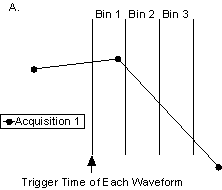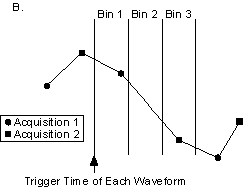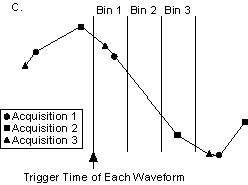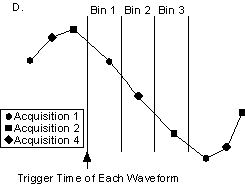# Equivalent-Time Sampling and Random Interleaved Sampling

NI High-Speed Digitizers Help (NI-SCOPE)

Edition Date: January 2017

Part Number: 370592AB-01

View Product InfoNI-SCOPE (English | Japanese)

Equivalent-time sampling is a sampling method in which a picture of a waveform is created over time by using a series of samples taken from repetitive waveforms.

Random Interleaved Sampling (RIS) is a form of equivalent-time sampling that increases apparent sample rates of repetitive signals by combining several triggered waveforms. Because the trigger time occurs randomly between two samples, the digitizer samples different points in the waveform on consecutive acquisitions. By combining these waveforms, you can achieve RIS sample rates that are up to 25 times higher than the ADC sample rate on the digitizer.

## How RIS Works

Each waveform trigger occurs at some time randomly distributed between two samples. The digitizer can measure the time from the trigger to the next sample, commonly called the time-to-digital conversion (TDC), extremely accurately—with hundreds of times more resolution than the sample period of the digitizer. With these TDC measurements, NI-SCOPE can combine multiple waveforms, aligned at the trigger time, to simulate a faster sampling rate for repetitive signals.

## How Oversampling Factors Increase Effective Sample Rates

When a digitizer is in RIS mode, the legal sample rates become multiples of the maximum real-time sampling rate. These multiples are the oversampling factor. For example, the NI 5112 has a 100 MS/s maximum real-time sampling rate, so the RIS sampling rates are the oversampling factor times 100 MS/s, where the oversampling factor is two, three, four, and so on. If you specify 300 MS/s for your sampling rate, the oversampling factor is three.

Each TDC value is between zero and the sampling period, so a 100 MS/s digitizer has TDC values between 0 and 10 ns. This time span is divided into a number of bins equal to the oversampling factor. For example, an oversampling factor of three means there is one TDC bin from 0 to 3.33 ns, another from 3.33 to 6.67 ns, and another from 6.67 to 10 ns. To reconstruct a waveform, each bin must contain at least one TDC value.

The following four figures show an example RIS acquisition with an oversampling factor of three. Each of the figures shows the aligned trigger time of the waveforms followed by the three TDC bins, where the combined width of the three bins is the real-time sample period of the digitizer.

1. The first waveform has a TDC value that falls in bin number 1.2. The succeeding waveform falls in bin number 3.3. Because of the randomness of the TDC value, another sampled waveform falls in bin 1. NI-SCOPE does not fetch this waveform from the digitizer because the bin is already filled.4. The next waveform falls in bin 2.Now all bins are full, so NI-SCOPE returns the three waveforms sampled at the maximum real-time rate as one evenly-sampled waveform with a sampling rate three times greater.